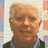How to Mass Create Records in a Sub-Table.

I now have packages arriving internationally and have found that they may have several tracking numbers as they move through various countries and/or carriers.  I can no longer keep up with this using one field and have created a sub-table for this purpose.

I have transaction 1327 records that I would like to create in the sub-table, moving the carrier and tracking number from the transaction table into the sub-table.

I need to take data from 2 fields in a table, create records in a subtable and put the data there. I'm encountering situations where packages that travel internationally have multiple tracking numbers and now need a subtable, not a single field, to keep them straight.`let t := (select ParentTableName);`
`let n := 0;`
`let c := null;`
`let s := null;`
`for i in t do`
`n := n + 1;`
`c := i.Field1;`
`s := i.Field2;`
`let p := (create ChildTableName);`
`p.(Field1 := c);`
`p.(Field2 := s)`
`end`Place above code in button on parent table form.Use below code instead of the above code:

`let t := (select ParentTableName);`
`let n := 0;`
`for i in t do`
`n := n + 1;`
`let p := (create ChildTableName);`
`p.('Carrier Number' := i.'Carrier Number');`
`p.('Tracking Number' := i.'Tracking Number')`
`end`